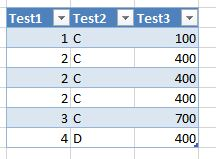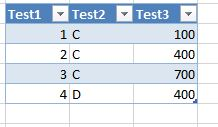# New to QlikView

Discussion board where members can get started with QlikView.Not applicable

## How we can use both Distinct and sum functions in set analysis

How we can use both Distinct and sum functions in set analysis?

See the below expression where i need to take the distinct values of the field Number before taking the Sum of Amount

=sum({<

Distinct(Number),

AAAAA={'C'}

>}Amount)

9 RepliesMVP

## Re: How we can use both Distinct and sum functions in set analysis

Sorry, I don't understand what you mean with " i need to take the distinct values of the field Number before taking the Sum".

Could you explain this with some lines of sample data and your expected outcome?MVP

## Re: How we can use both Distinct and sum functions in set analysis

Try:

sum({\$<Number= {'=COUNT(DISTINCT Number)'},AAAAA={'C'}>} Amount)Not applicable

## Re: How we can use both Distinct and sum functions in set analysis

Yeah Sure,

See the below examplehere i want the result ashere you can see like we are taking the distinct of Test1 and after that i want to sum the field Test3.Not applicable

## Re: How we can use both Distinct and sum functions in set analysis

Robert, Its not workingMVP

## Re: How we can use both Distinct and sum functions in set analysis

Maybe something like

=sum( aggr( only(Test3), Test1, Test2))

But I would rather look into your data model.Partner

## Re: How we can use both Distinct and sum functions in set analysis

Hi,

My suggestion is to move the calculation into the load script. Maybe you'll also need to calc count of such records, sum of some fields, etc. But you'll definitely avoid extra calculations in run-time.

Best regards,

MaximMVP

## Re: How we can use both Distinct and sum functions in set analysis

Did you try Stefan's solution or Maxim's suggestion?

If it still does not work and you this as Set Analysis - post your data+error+desired result.

Contributor

## Re: How we can use both Distinct and sum functions in set analysis

I simply added TEST1 and TEST 2 as dimensions into straight table and in Expression i used below and I could get what you described.

Sum(distinct Test3)

Valued Contributor